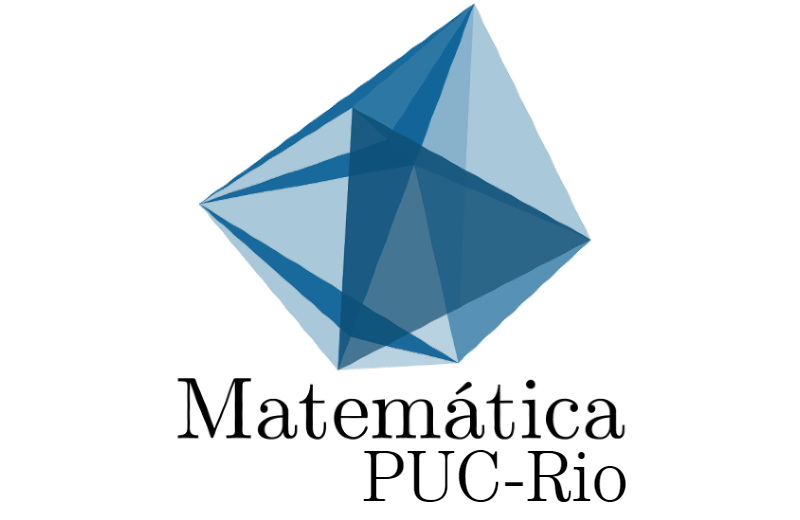SEMINÁRIO DE GEOMETRIA E TOPOLOGIA - The homotopy type of spaces of locally convex curves in the sphereThe homotopy type of spaces of locally convex curves in the sphere
Expositor: Nicolau Saldanha
Instituição: PUC Rio
Data e Horário: 20/04/18 | 14:00h

RESUMO: A smooth curve in the sphere is locally convex (or nondegenerate) if its Frenet frame is well defined. Thus, for instance, a curve in the 2-sphere is locally convex iff its geodesic curvature is nonvanishing (wlog positive); a curve in the 3-sphere is locally convex iff its torsion is nonvanishing. Spaces of locally convex curves in the sphere are not well understood, and this problem is related to many other areas of mathematics (including differential equations and control theory). In this talk we give a quick introduction to this subject, followed by some recent results. Includes joint work with E. Alves, V. Goulart, B. Shapiro, C. Zhou and P. Zuhlke.